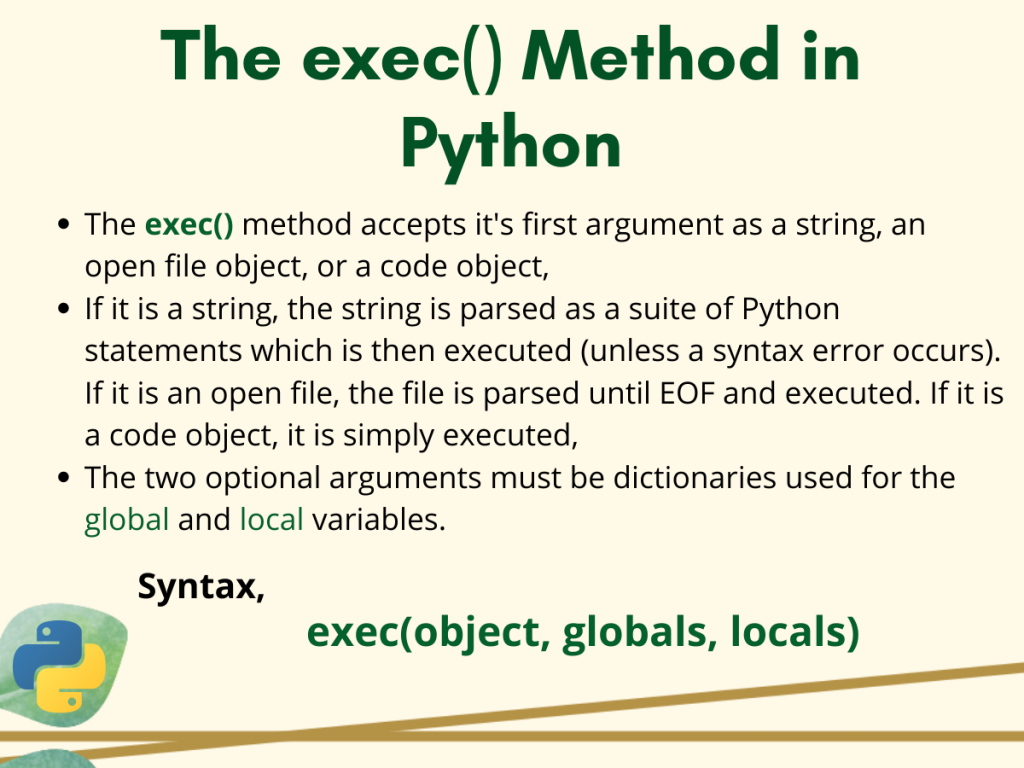# Understanding the Python exec() MethodSo today in this tutorial, let’s get to know about the Python exec() method in Python.

## The Python exec() Method

Basically, the Python `exec()` method executes the passed set of code in the form of string. It is very useful as it practically supports dynamic execution. The syntax for the method is given below.

```exec(object, globals, locals)
```

Here, `object` could be a string, an open file object, or a code object.

• For string – the string is parsed as a suite of Python statements which is then executed (unless a syntax error occurs).
• For an open file – the file is parsed until EOF and executed.
• For a code object – it is simply executed.

And the two optional arguments `globals` and `locals` must be dictionaries used for the global and local variables.

Now that we already have a basic idea of the `exec()` method, let us try to understand it’s working through an example.

```>>> exec("print('Hey!')")
Hey!
>>> exec("print(6+4)")
10
```

It is clear from the above code snippet, the `print()` statements are successfully executed by the `exec()` method and we get the desired results.

## Working with the Python exec() Method

Now let us jump right into some examples exploring how the `exec()` method works in Python with and without the `globals` and `locals` parameters.

### 1. Without Global and local Parameters

In the previous example, we simply executed some set of instructions in Python passing the object argument to the `exec()` method. But, we didn’t see the names in the current scope.

Now let us use the dir() method to get the list of current methods and names with `math` module included before calling the `exec()` method.

```from math import *

exec("print(pow(2, 5))")

exec("print(dir())")
```

Output:

```32.0
['__annotations__', '__builtins__', '__cached__', '__doc__', '__file__', '__loader__', '__name__', '__package__', '__spec__', 'acos', 'acosh', 'asin', 'asinh', 'atan', 'atan2', 'atanh', 'ceil', 'copysign', 'cos', 'cosh', 'degrees', 'e', 'erf', 'erfc', 'exp', 'expm1', 'fabs', 'factorial', 'floor', 'fmod', 'frexp', 'fsum', 'gamma', 'gcd', 'hypot', 'inf', 'isclose', 'isfinite', 'isinf', 'isnan', 'ldexp', 'lgamma', 'log', 'log10', 'log1p', 'log2', 'modf', 'nan', 'pi', 'pow', 'radians', 'remainder', 'sin', 'sinh', 'sqrt', 'tan', 'tanh', 'tau', 'trunc']
```

As you can see, the various methods including `builtins`, as well as from the `math` module is right now in the current scope and available to the Python `exec()` method.

This raises a big security issue when thinking of executing dynamic Python code. A user may include some modules to access system commands that even can crash your computer. Using the `globals` and `locals` parameters we can literally restrict the `exec()` to go beyond the methods that we want to access.

### 2. With globals parameter

Now let us see how we can use the Python `exec()` method with the globals parameter. Python allows us to pass and specify only the methods that we want the `exec()` method to access(in the form of a dictionary) from the builtin module.

```def squareNo(a):
return a*a

exec('print(squareit(10))',{"__builtins__":{"squareit": squareNo, "print": print}})

exec("print(dir())")
```

Output:

```100
['__annotations__', '__builtins__', '__cached__', '__doc__', '__file__', '__loader__', '__name__', '__package__', '__spec__', 'squareNo']
```

In the code above, we have passed a dictionary containing the methods `squareNo()` (mapped to a custom name squareit) and `print()`. Note, using any other method from the builtin method would raise a `TypeError`.

### 3. With locals parameter

When we only pass the `local` parameter(dictionary), by default all the builtin methods are also made available until and unless we explicitly exclude them.

Look at the example below, here though we have specified the `locals` dictionary all the builtin and math module methods are available in the current scope.

```from math import *
def squareNo(a):
return a*a

#global And local parameters
exec('print(pow(4,3))', {"squareit": squareNo, "print": print})

exec("print(dir())")
```

Output:

```64
['__annotations__', '__builtins__', '__cached__', '__doc__', '__file__', '__loader__', '__name__', '__package__', '__spec__', 'acos', 'acosh', 'asin', 'asinh', 'atan', 'atan2', 'atanh', 'ceil', 'copysign', 'cos', 'cosh', 'degrees', 'e', 'erf', 'erfc', 'exp', 'expm1', 'fabs', 'factorial', 'floor', 'fmod', 'frexp', 'fsum', 'gamma', 'gcd', 'hypot', 'inf', 'isclose', 'isfinite', 'isinf', 'isnan', 'ldexp', 'lgamma', 'log', 'log10', 'log1p', 'log2', 'modf', 'nan', 'pi', 'pow', 'radians', 'remainder', 'sin', 'sinh', 'sqrt', 'squareNo', 'tan', 'tanh', 'tau', 'trunc']
```

Hence, now explicitly excluding the builtins.

```from math import *

def squareNo(a):
return a*a

#explicitly excluding built-ins
exec('print(pow(4,3))', {"__builtins__": None},{"squareit": squareNo, "print": print})

exec("print(dir())")
```

Output:

```Traceback (most recent call last):
File "C:/Users/sneha/Desktop/test.py", line 7, in <module>
exec('print(pow(4,3))', {"__builtins__": None},{"squareit": squareNo, "print": print})
File "<string>", line 1, in <module>
TypeError: 'NoneType' object is not subscriptable
```

In the code above, restricting the `exec()` method for using only the passed(locals) methods practically makes the `pow()` method inaccessible. Hence, while running it we get the `TypeError`.

## exec() VS eval() in Python

There are two major differences between the `eval()` and `exec()` methods even though nearly do the same job.

1. eval() can execute only one expression whereas exec() can be used for executing a dynamically created statement or program which can include loops, `if-else` statements, function and `class` definitions,
2. eval() returns the value after executing a particular expression whereas exec() basically returns nothing and simply ignores the value.

## Conclusion

So that’s it for today. Hope you had a clear understanding of the working as well use of the Python `exec()` method.

For any further Python `exec()` related questions, feel free to ask in the comments below.